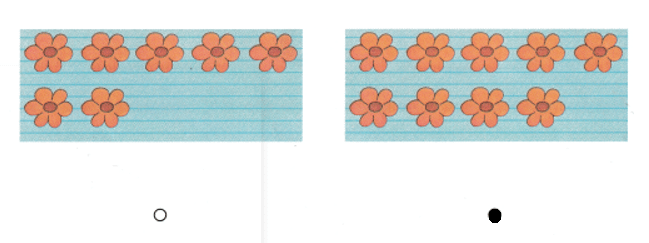# Texas Go Math Kindergarten Lesson 6.4 Answer Key One More and One Less

Refer to our Texas Go Math Kindergarten Answer Key Pdf to score good marks in the exams. Test yourself by practicing the problems from Texas Go Math Kindergarten Lesson 6.4 Answer Key One More and One Less.

## Texas Go Math Kindergarten Lesson 6.4 Answer Key One More and One Less

Explore

DIRECTIONS: Place 8 counters in the ten frame. Take one counter away. Write the number that is one less than 8. Place 8 counters in the ten frame again. Add one more counter. Write the number that is one more than 8.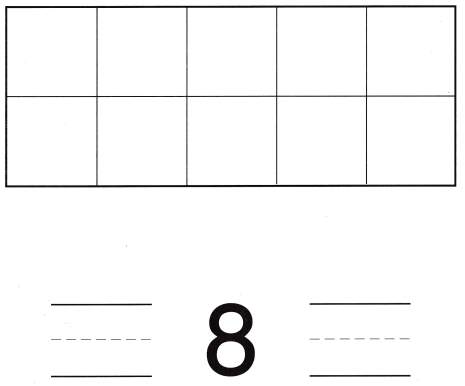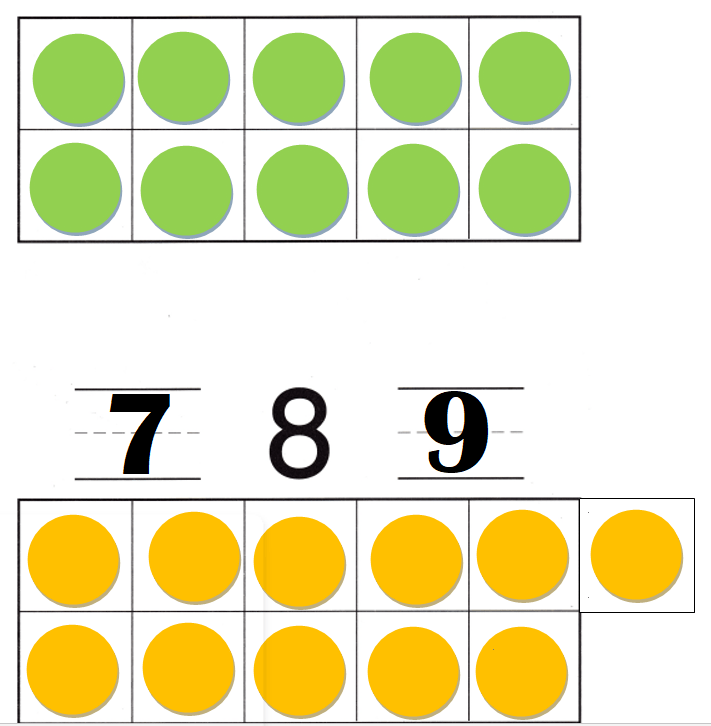Explanation:
One less than 8 = 8 – 1 = 7.
One counter added to 8 = 8 + 1 = 9.

Share and Show

DIRECTIONS: 1. There are 6 erasers in the set. Write the number that is one less than 6. Write the number that is one more than 6. 2. There are 3 crayons in the set. Write the number that is one less than 3. Write the number that is one more than 3.

Question 1.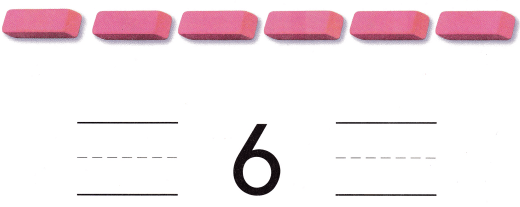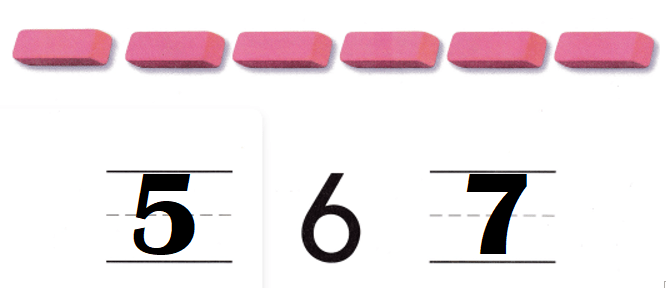Explanation:
One less than 6 = 6 – 1 = 5.
One counter added to 6 = 6 + 1 = 7.

Question 2.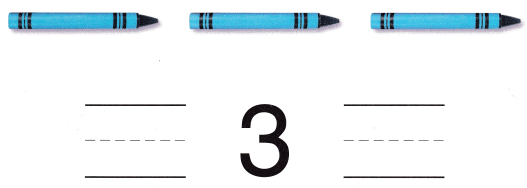Explanation:
One less than 3 = 3 – 1 = 2.
One counter added to 3 = 3 + 1 = 4.

DIRECTIONS: 3-5. Look at the number. Write the number that is one less. Write the number that is one more.

Question 3.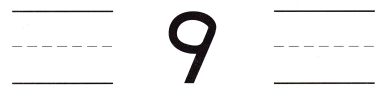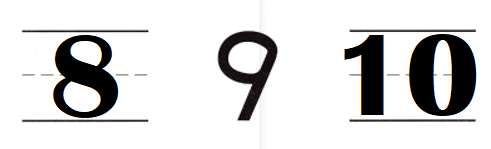Explanation:
One less than 9 = 9 – 1 = 8.
One counter added to 9 = 9 + 1 = 10.

Question 4.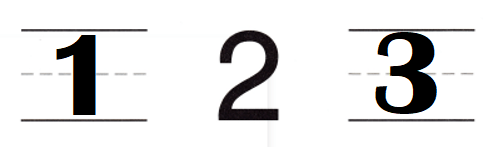Explanation:
One less than 2 = 2 – 1 = 1.
One counter added to 2 = 2 + 1 = 3.

Question 5.Explanation:
One less than 4 = 4 – 1 = 3.
One counter added to 4 = 4 + 1 = 5.

HOME ACTIVITY • Show your child a set of seven household objects. Have your child create a set of objects that has one more object than your set.
Yes, he counted all and said 7 cars + 1 truck = 8 toys.

Explanation:
I have given seven different types of cars to him..
He counted and bought one more truck.
He counted all and said 7 cars + 1 truck = 8 toys.

DIRECTIONS: 6. Gabe has 5 hats. Chloe has one more hat than Gabe. How many hats does Chloe have? Draw Chloe’s hats. Write the number. 7. Jack has 3 marbles. Pat has one more marble than Jack. Draw Pat’s marbles and write how many.

Problem Solving
Question 6.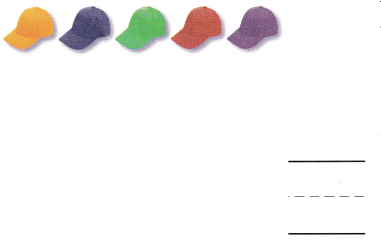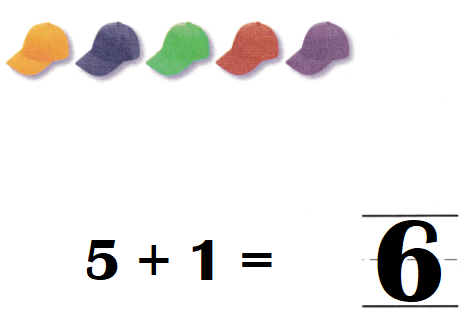Explanation:
Number of hats Gabe has = 5.
Chloe has one more hat than Gabe.
=> Number of hats Chole has = Number of hats Gabe has + 1
= 5 + 1
= 6.

Question 7.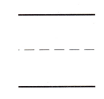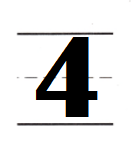Explanation:
Jack has 3 marbles.
Number of marbles Jack has = 3.
Pat has one more marble than Jack.
Number of marbles Pat has = Number of marbles Jack has + 1
= 3 + 1
= 4.

### Texas Go Math Kindergarten Lesson 6.4 Homework and Practice Answer Key

DIRECTIONS: 1. There are 7 pencils in the set. Write the number that is one less than 7. Write the number that is one more than 7. 2-3. Look at the number. Write the number that is one less. Write the number that is one more.

Question 1.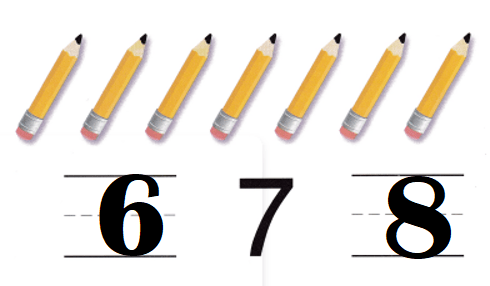Explanation:
One less than 7 = 7 – 1 = 6.
One more than 7 = 7 + 1 = 8.

Question 2.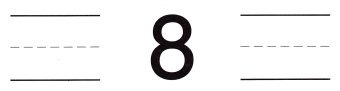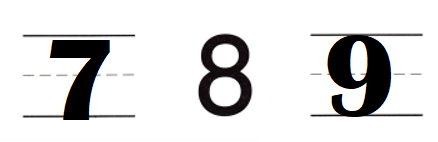Explanation:
One less than 8 = 8 – 1 = 7.
One more than 8 = 8 + 1 = 9.

Question 3.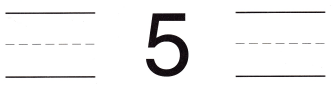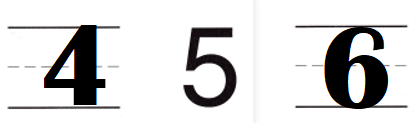Explanation:
One less than 7 = 7 – 1 = 6.
One more than 7 = 7 + 1 = 8.

DIRECTIONS: Choose the correct answer. 4. Jessica has o sticker with 4 stars. Which sticker has 1 more star? 5. Beth has a picture of 10 flowers. Which picture has a number of flowers that is 1 less than 10?

Lesson Check
Question 4.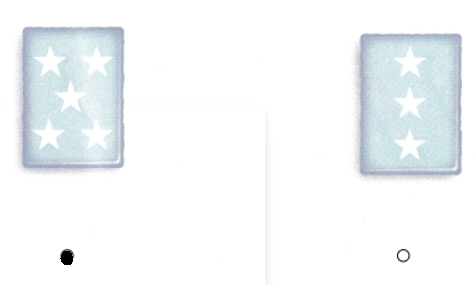Explanation:
Jessica has o sticker with 4 stars.
Which sticker has 1 more star = 4 + 1 = 5.

Question 5.